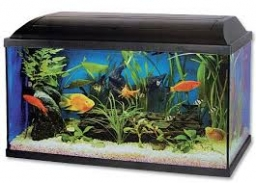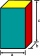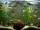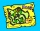# Aquarium height

How high does the water in the aquarium reach, if there are 36 liters of water in it? The length of the aquarium is 60 cm and the width is 4 dm.

c =  1.5 dm

### Step-by-step explanation:Did you find an error or inaccuracy? Feel free to write us. Thank you!Tips to related online calculators
Do you know the volume and unit volume, and want to convert volume units?

## Related math problems and questions:

• AquariumCan 30 liters of water fit in a cuboid aquarium with dimensions a = 3dm b = 6dm c = 5dm?
• Cuboid aquariumCuboid 25 times 30 cm. How long is third side if cuboid contains 30 liters of water?
• The aquariumThe aquarium is 120 cm long, 70 cm wide and 96 cm high. How high does the water reach if they filled 168l of water into the aquarium?
• Aquarium 6How high is the water level in the aquarium with a rectangular base 40cm and 50cm if it is filled 0,65hl of water?
• Empty aquariumHow much does an empty aquarium weigh with dimensions: length = 40 cm, width = 30 cm, height = 20 cm, if 1 dm2 of glass weighs 300 g? Calculate its weight in kilograms.
• Water tankWhat is the height of the cuboid-shaped tank with the bottom dimensions of 80 cm and 50 cm if the 480 liters of water reach 10 cm below the top?
• Water in aquariumThe aquarium cuboid shape with a length of 25 cm and a width of 30 cm is 9 liters of water. Calculate the areas which are wetted with water.
• AquariumHow many liters of water can fit in an aquarium measuring 30, 15 and 20 cm?
• AquariumFind how many dm2 of glass we need to make a block-shaped aquarium (the top is not covered) if the dimensions of the aquarium are to be: width 50 cm, length 120 cm, and height 8.5 dm.
• Edges of the cuboidFind the length of the edges of the cuboid, which has the following dimensions: width is 0.4 m; the height is 5.8 dm and the block can hold 81.2 liters of fluid.
• Liters in cylinderDetermine the height at which level 24 liters of water in a cylindrical container having a bottom diameter 36 cm.
• For thinkingsThe glass cube dive into the aquarium, which has a length of 25 cm, width 20 cm and height of 30 cm. Aquarium water rises by 2 cm. a) What is the volume of a cube? b) How many centimeters measure its edge?
• Water tank300hl of water was filled into the tank 12 m long and 6 m wide. How high does it reach?
• SnailsHow many liters of water will fit in an aquarium with bottom dimensions of 30 cm and 25 cm and a height of 60 cm, if we pour water up to a height of 58 cm? How many most snails can we keep in an aquarium, if we know that snails need 600 cm ^ 3 of water fo
• Cubes into cuboidHow many 12 centimeter cubes fit into the block (cuboid) with 6dm, 8.4dm, and 4.8?
• Plan scaleThe actual width of the room is 66 dm, the width in plan is 4.4 cm. What scale corresponds to the plan?
• Swimming pool 4The pool shaped cuboid measuring 12.5 m × 640 cm at the bottom is 960hl water. To what height in meters reaches the water level?